# 02.22.09

## Why You Invest When the Market Goes Down

Posted in finance, math at 2:51 pm by danvk

The S&P 500 certainly hasn’t made anyone rich over the last year: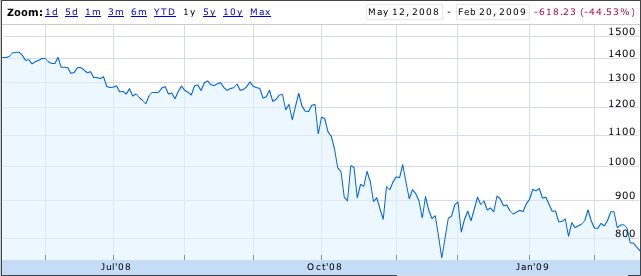Most people see this and think “an investment one year ago would have lost 45% of its value”. Others think “great, now stocks are all cheaper!”

In reality, most ordinary people invest portions of their paychecks, either through their 401(k) or a personal account. This means they’re doing time averaging. Sure, investments when the market was up aren’t doing well. But investments when the market was down are doing just fine.

This is all kind of wishy-washy, though. Let’s try to quantify it. Suppose a market drop and recovery looks like a parabola: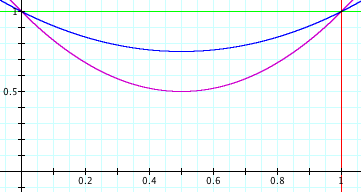The prices here are parabolas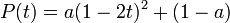$P(t) = a(1-2t)^2 + (1-a)\,$

for various values of a. a=0 means the market is flat. a=0.5 means the market loses 50% of its value.

If you invest dt dollars in this market at each point in time, you can work out a nasty integral and show that the number of shares you have at time t is: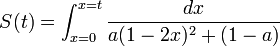$S(t) = \int_{x=0}^{x=t} \frac{dx}{a(1-2x)^2+(1-a)}$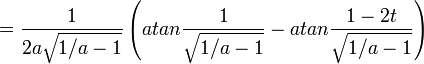$= \frac{1}{2a \sqrt{1/a - 1}} \left(atan \frac{1}{\sqrt{1/a - 1}} - atan \frac{1-2t}{\sqrt{1/a - 1}} \right)$

and hence the value of your shares at the end is: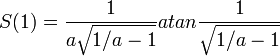$S(1) = \frac{1}{a \sqrt{1/a - 1}} atan \frac{1}{\sqrt{1/a - 1}}$

Here’s what that looks like: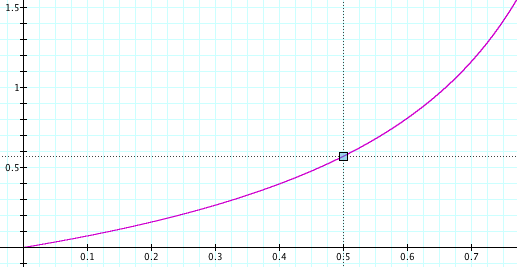The x-axis is a, the fraction by which the market drops. The y-axis is your total return on investment. If the market drops by 50% (a=0.5) then your total return on investment is around 55%. With time-averaging, the more the market drops, the better you do.

This makes a lot of sense if you think about it. Say the market drops 99.9% and then recovers. The shares you bought when it was at its bottom earned you a return of 1000x. Investing at the bottom is important! You should keep investing, even as the market drops. If you don’t, you’ll miss that bottom.